# R S Aggarwal Solutions for Class 10 Maths Chapter 11 T-Ratios of Some Particular Angles

R S Aggarwal Class 10 Solutions for Chapter 11 T-Ratios of Some Particular Angles are available here. This chapter is composed of only one exercise which includes concepts like trigonometric ratios of different angles (45 degrees, 60 degrees and 30 degrees) and axioms for T-ratios. The solutions created are genuine and can be referred by the students to prepare for their Maths exam. The set of questions and answers helps students to master T-Ratios.

## Download PDF of R S Aggarwal Solutions for Class 10 Chapter 11 T-Ratios of Some Particular Angles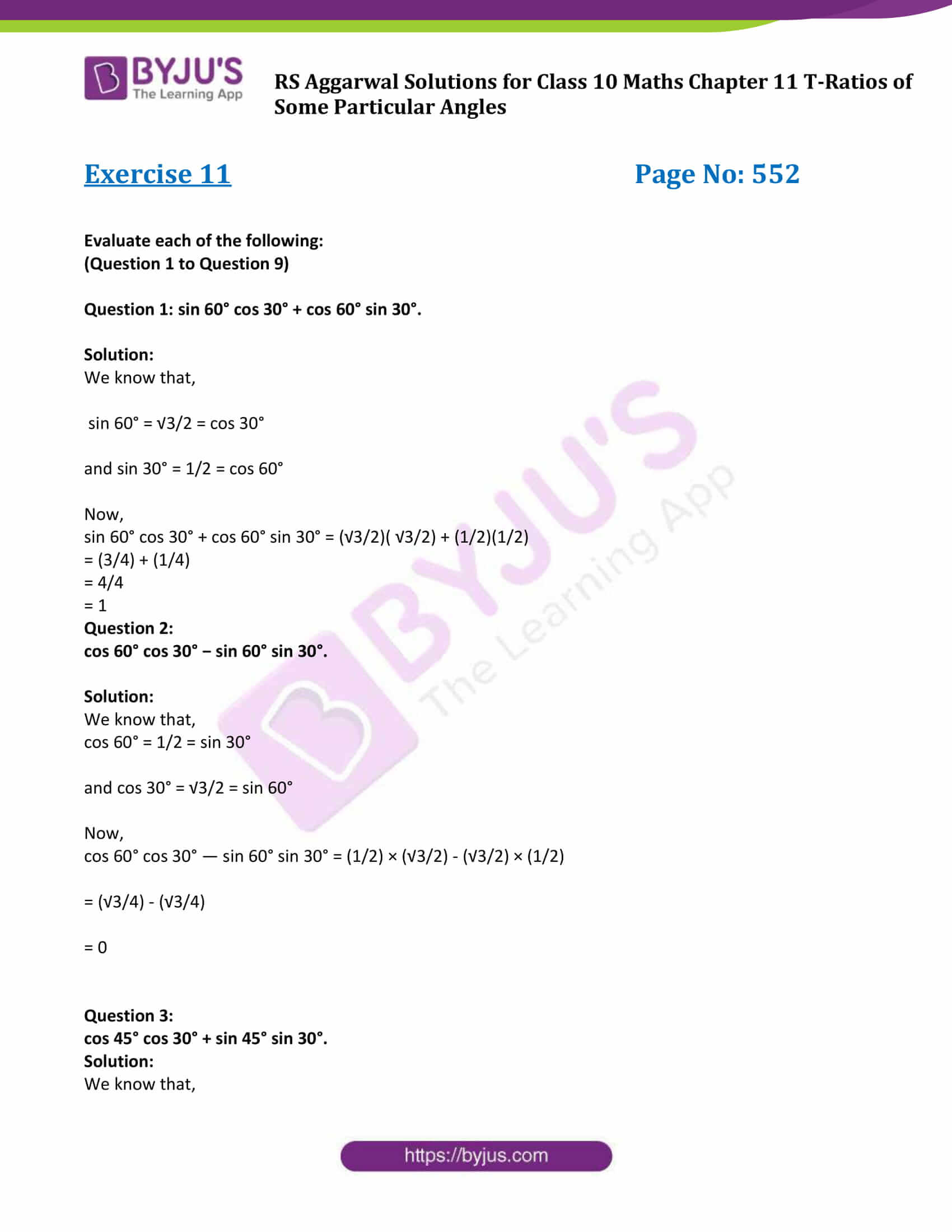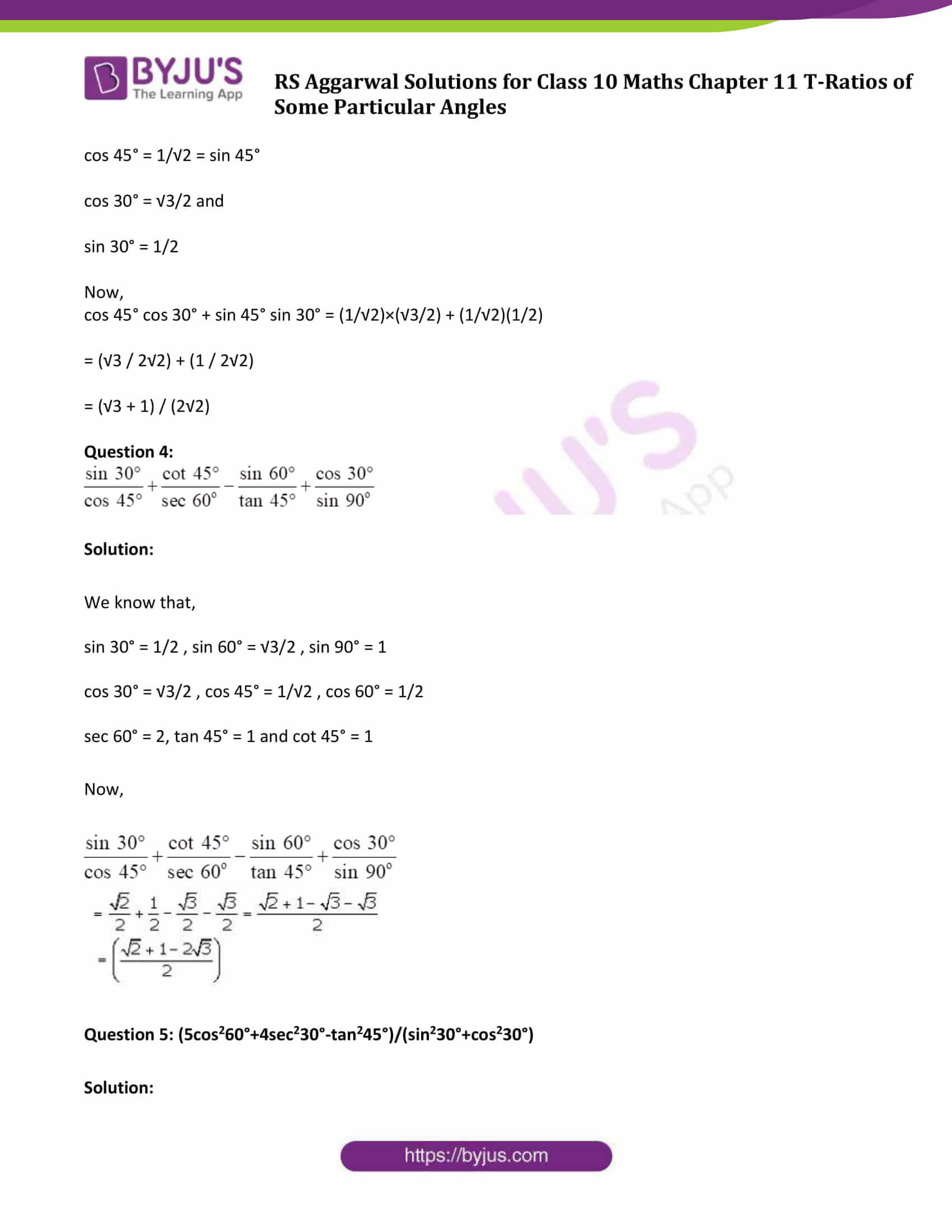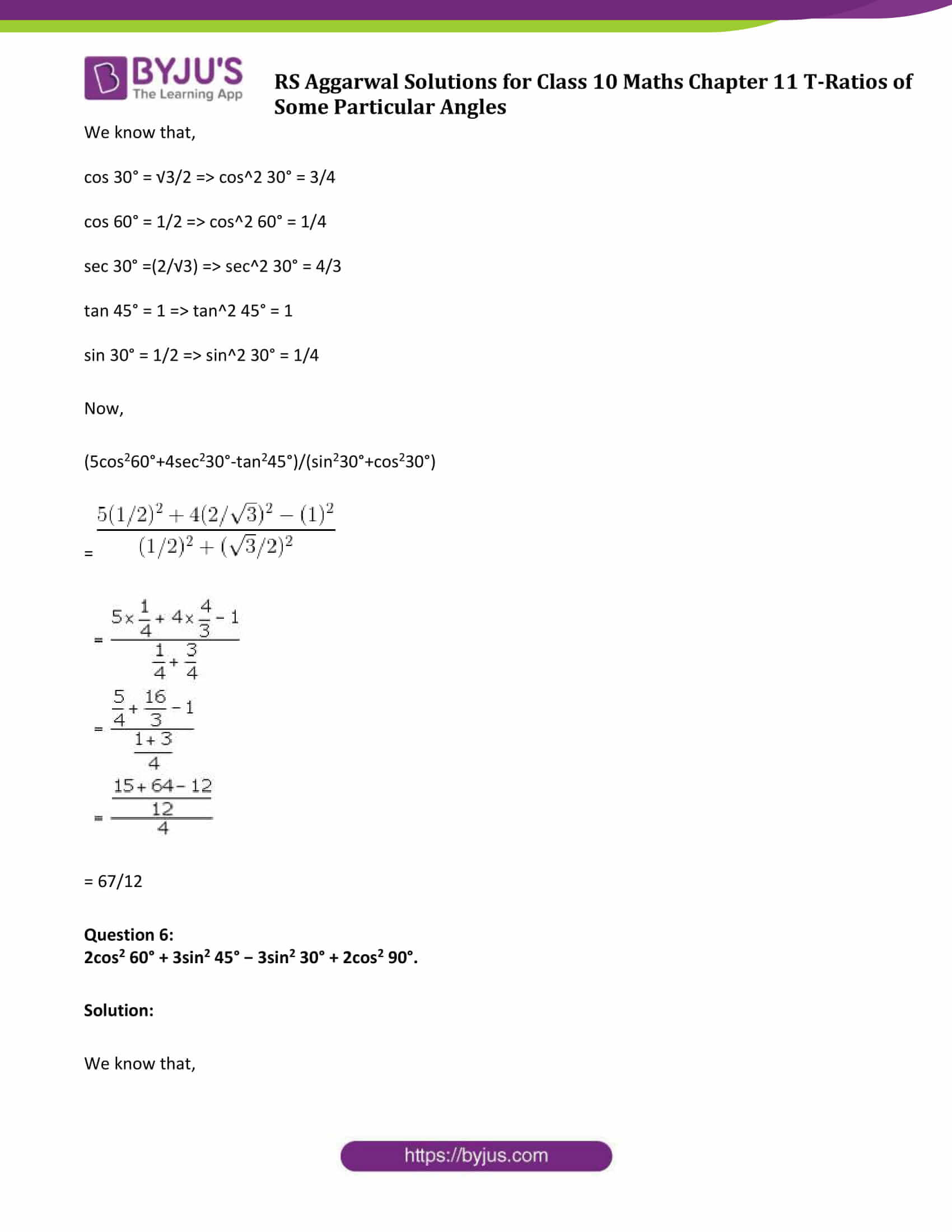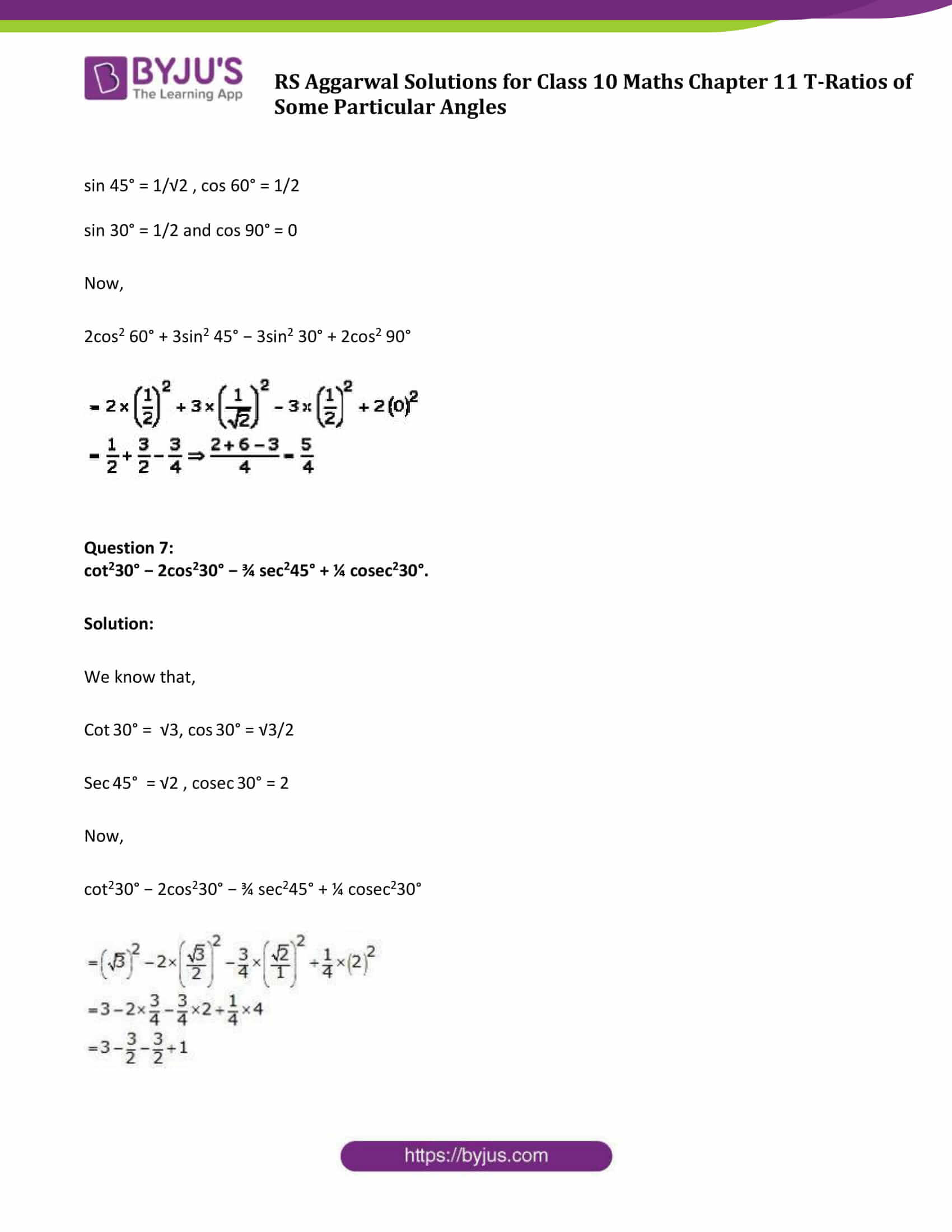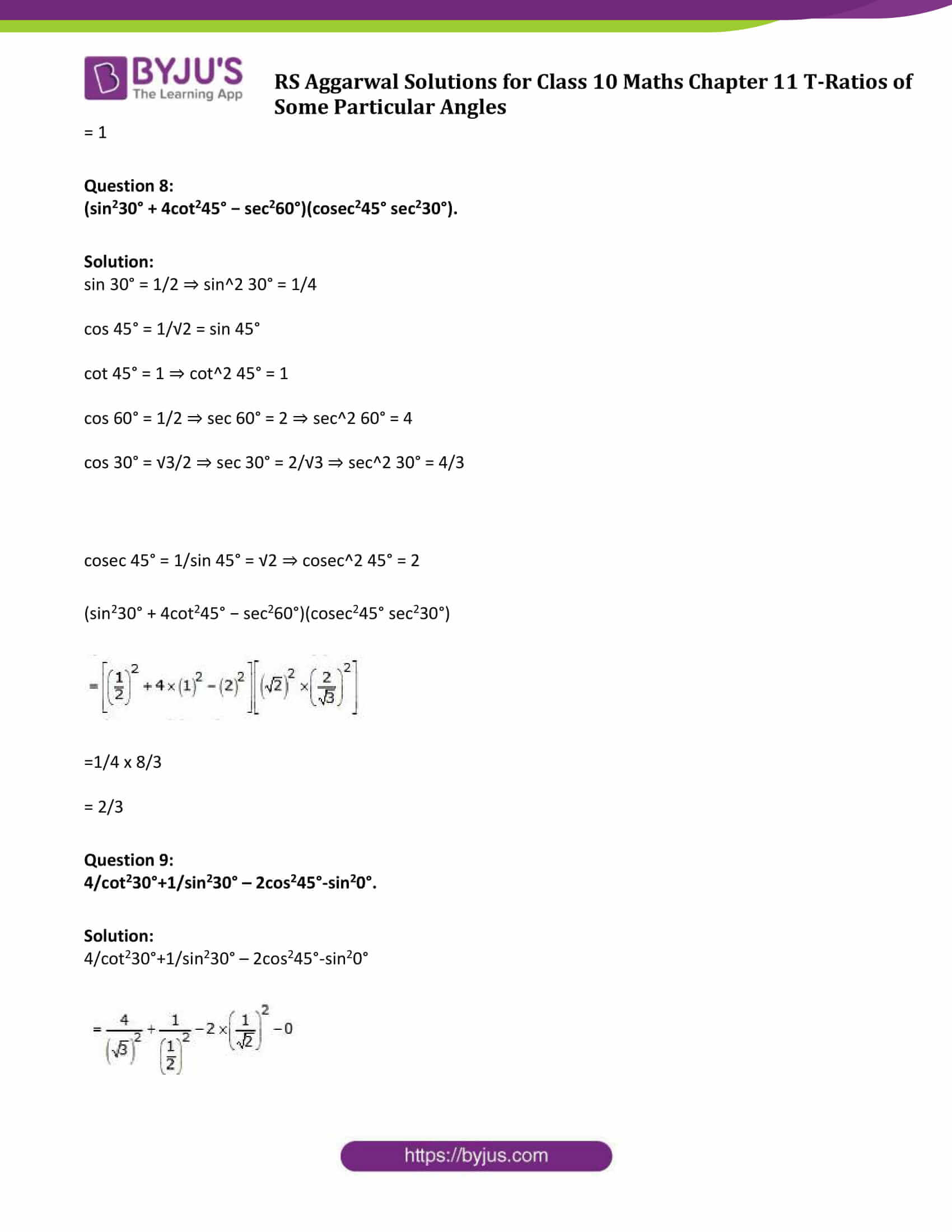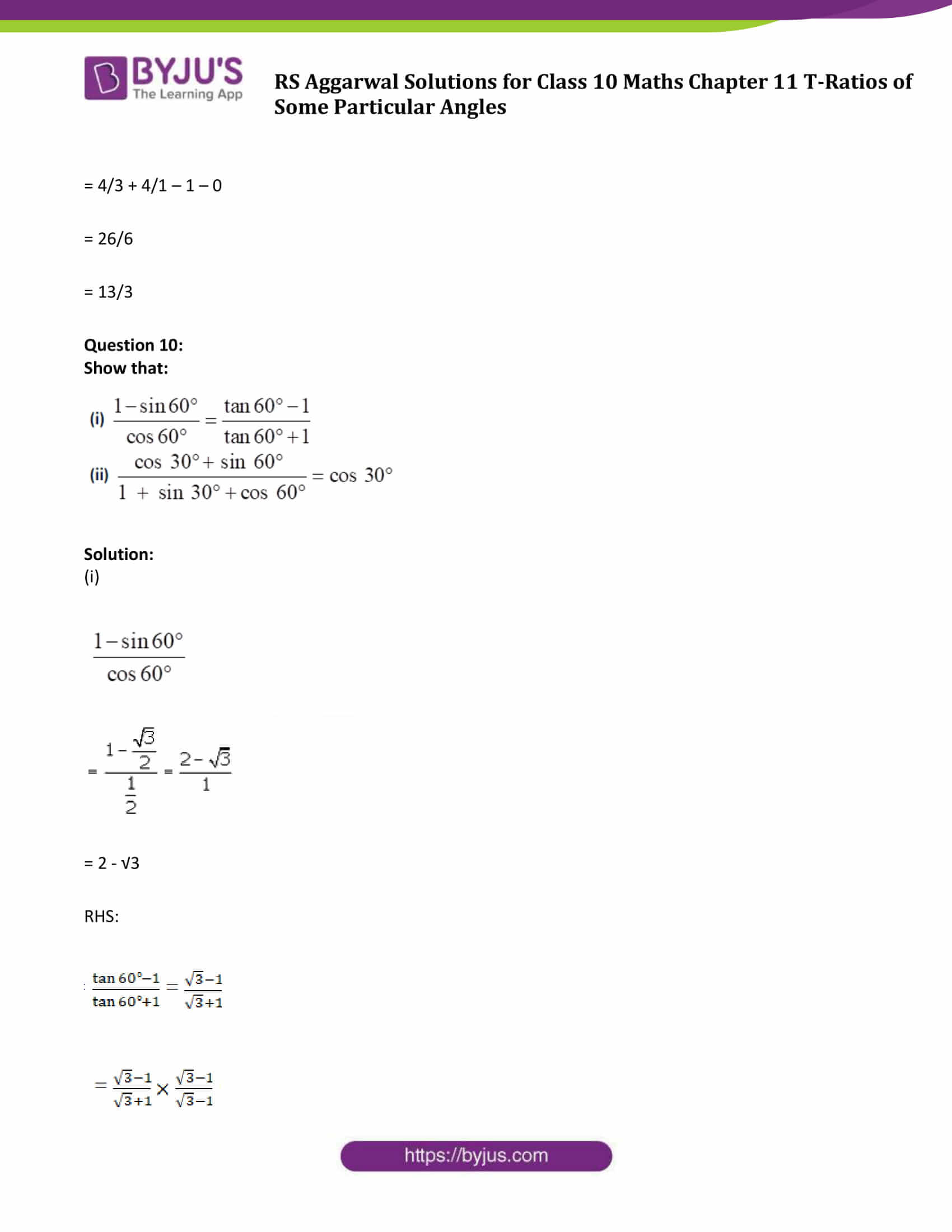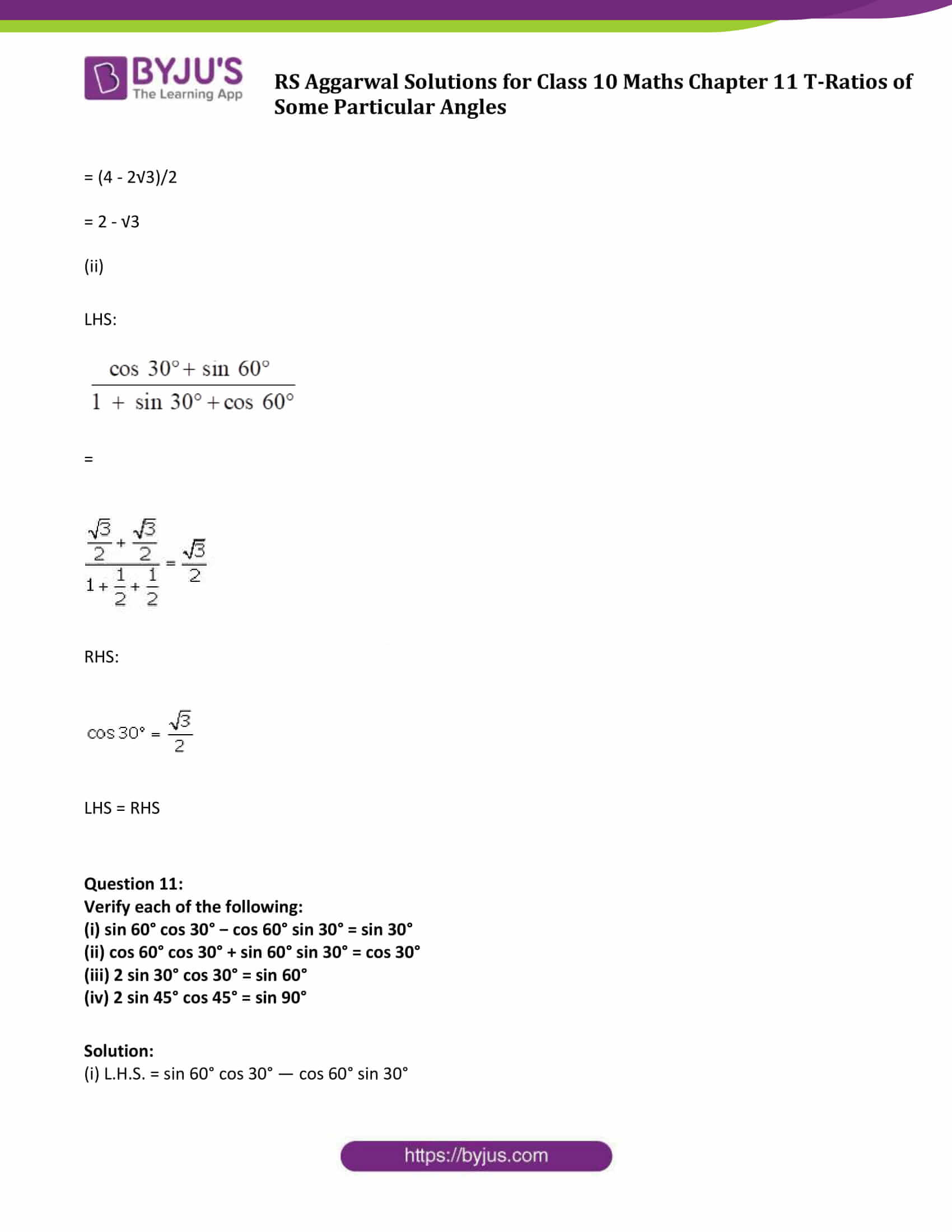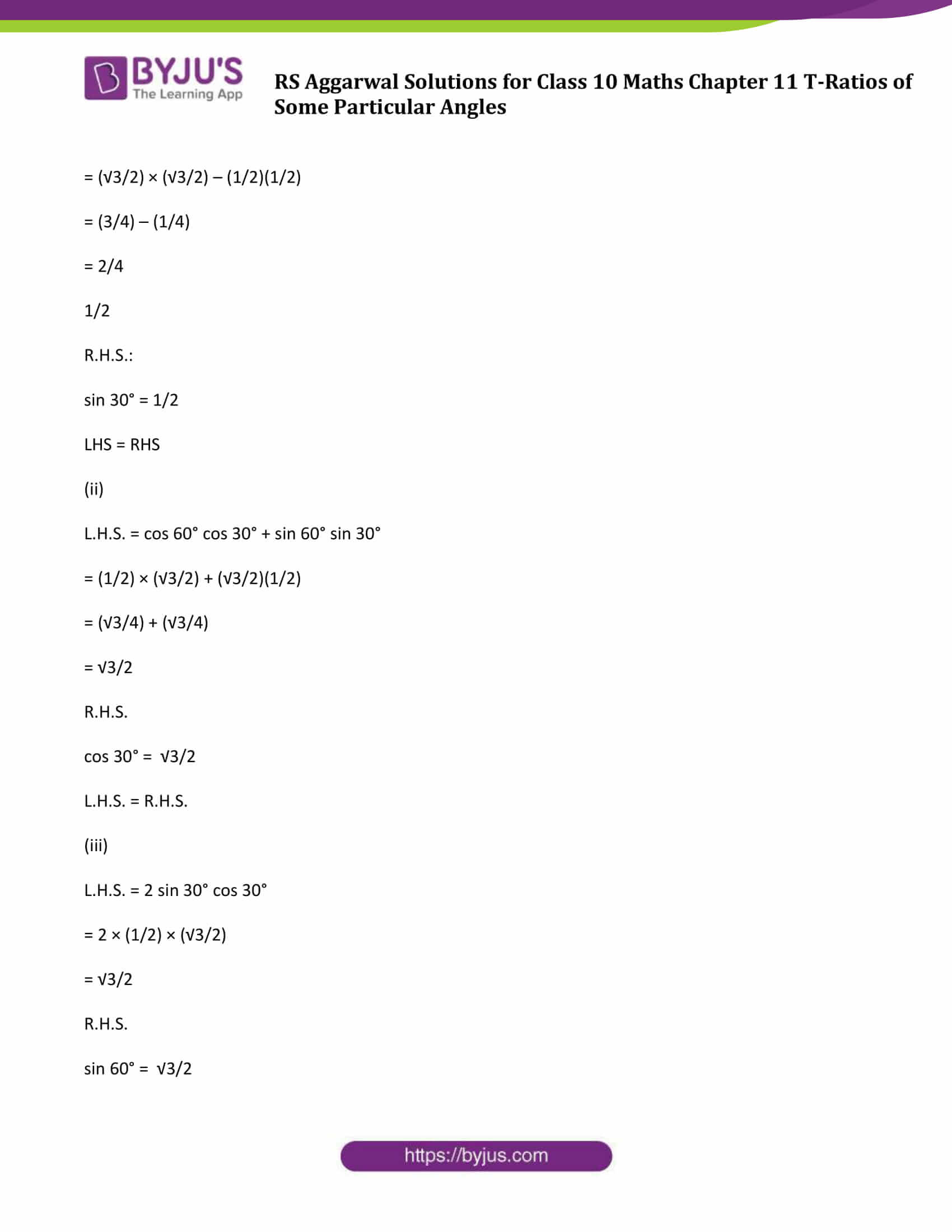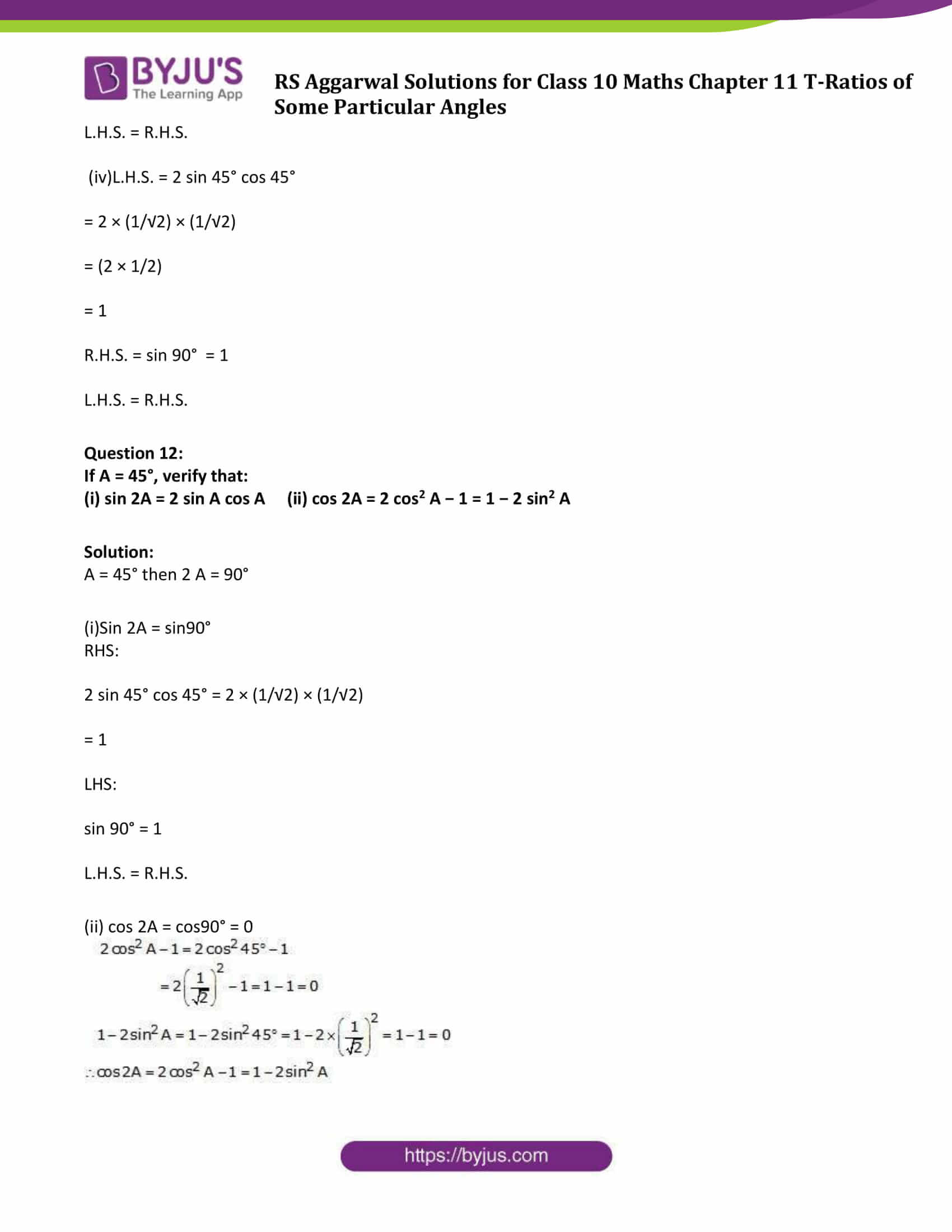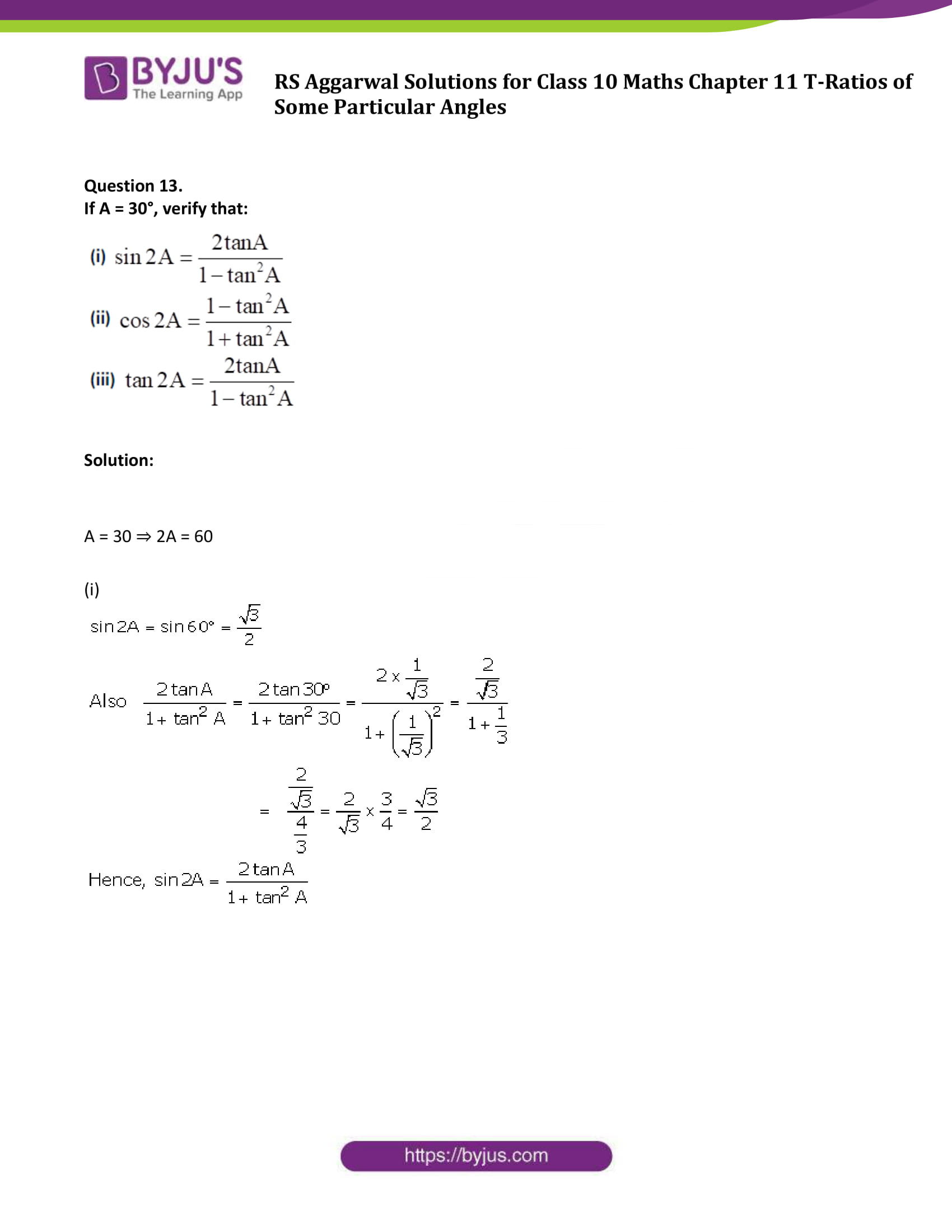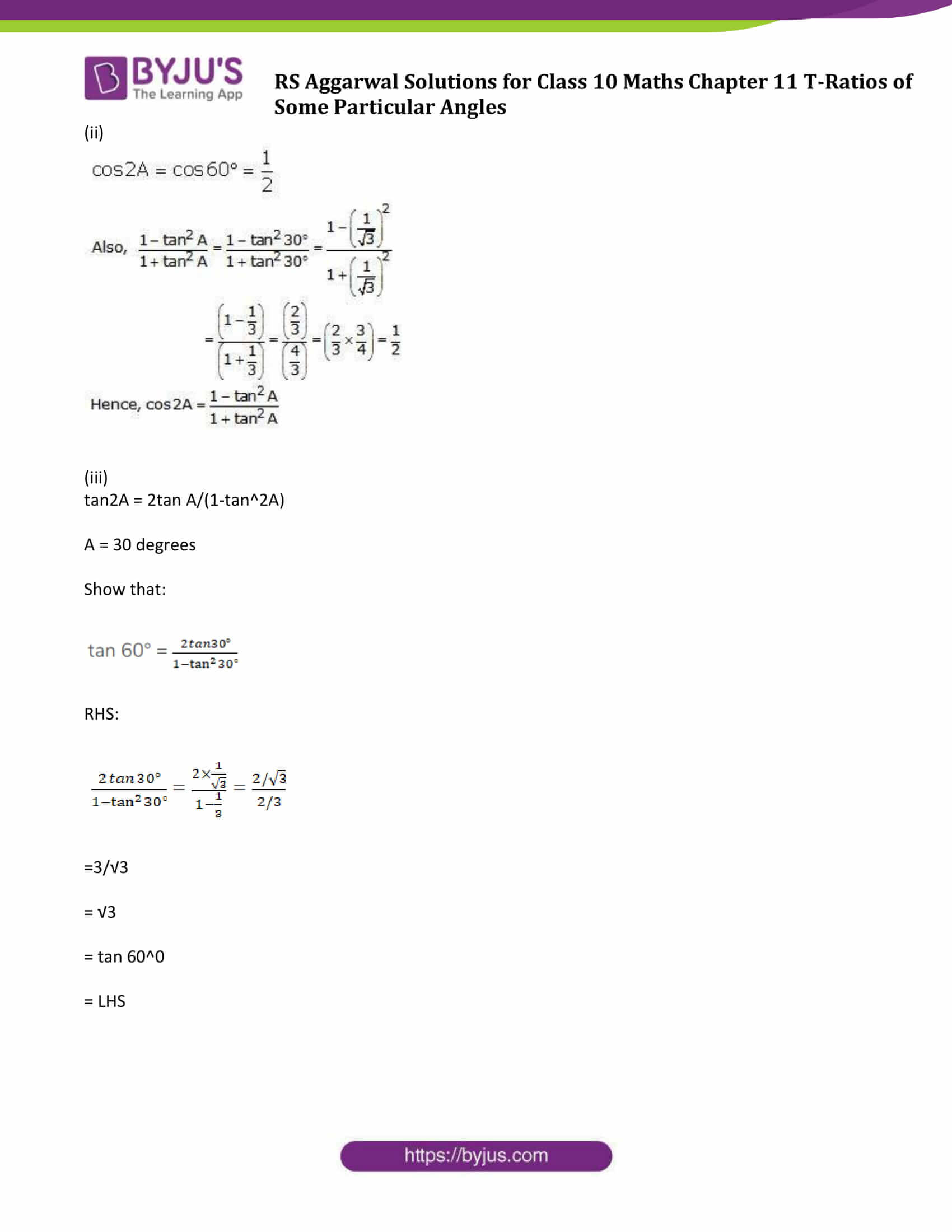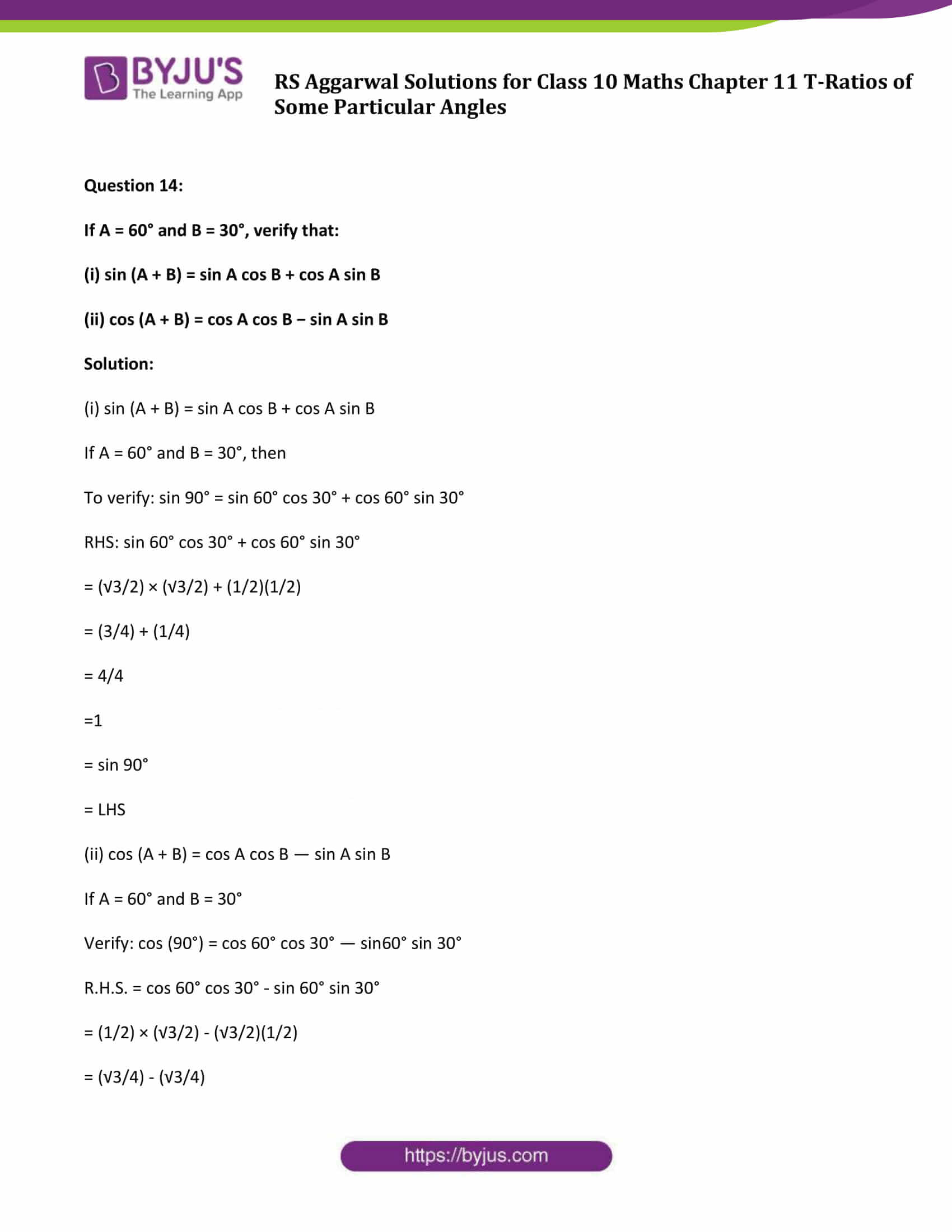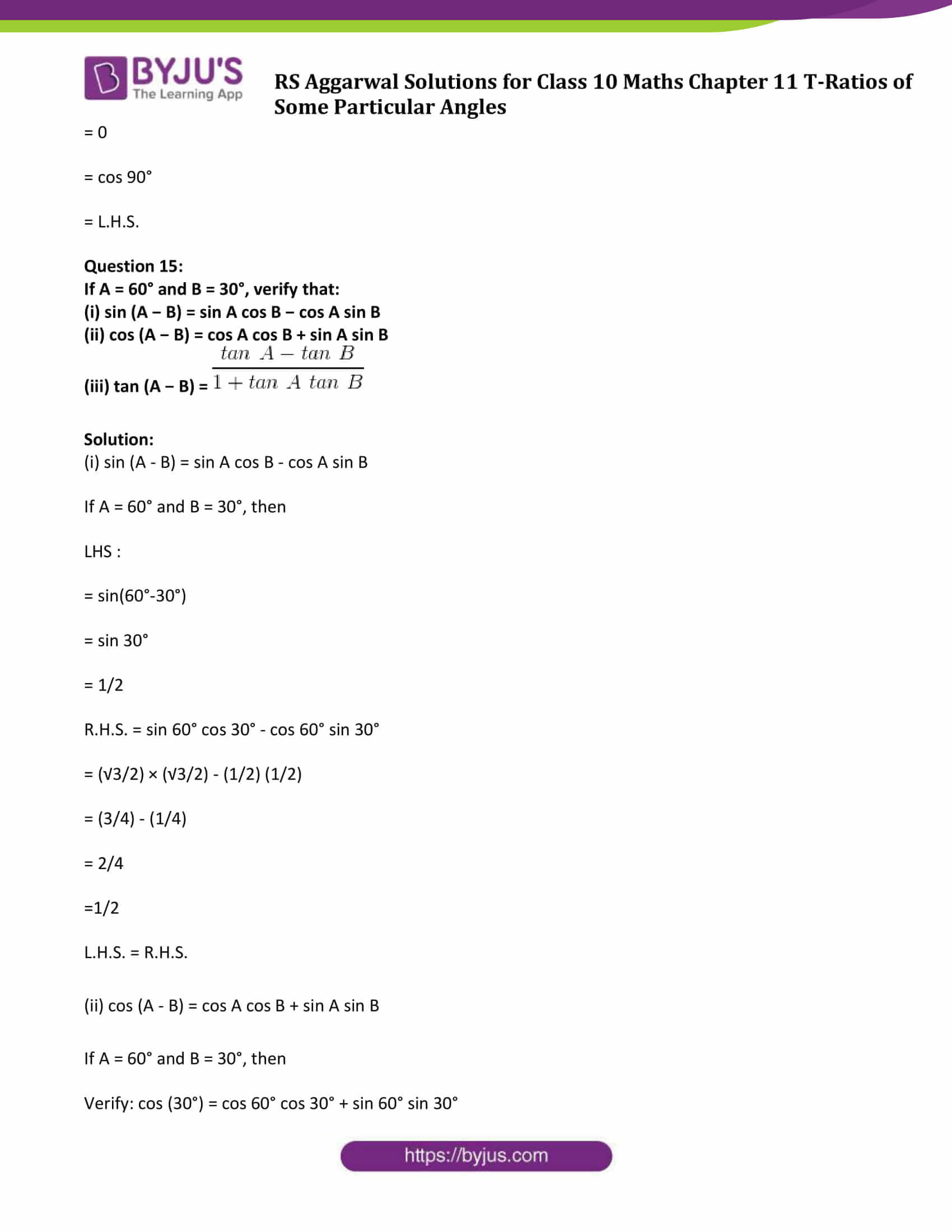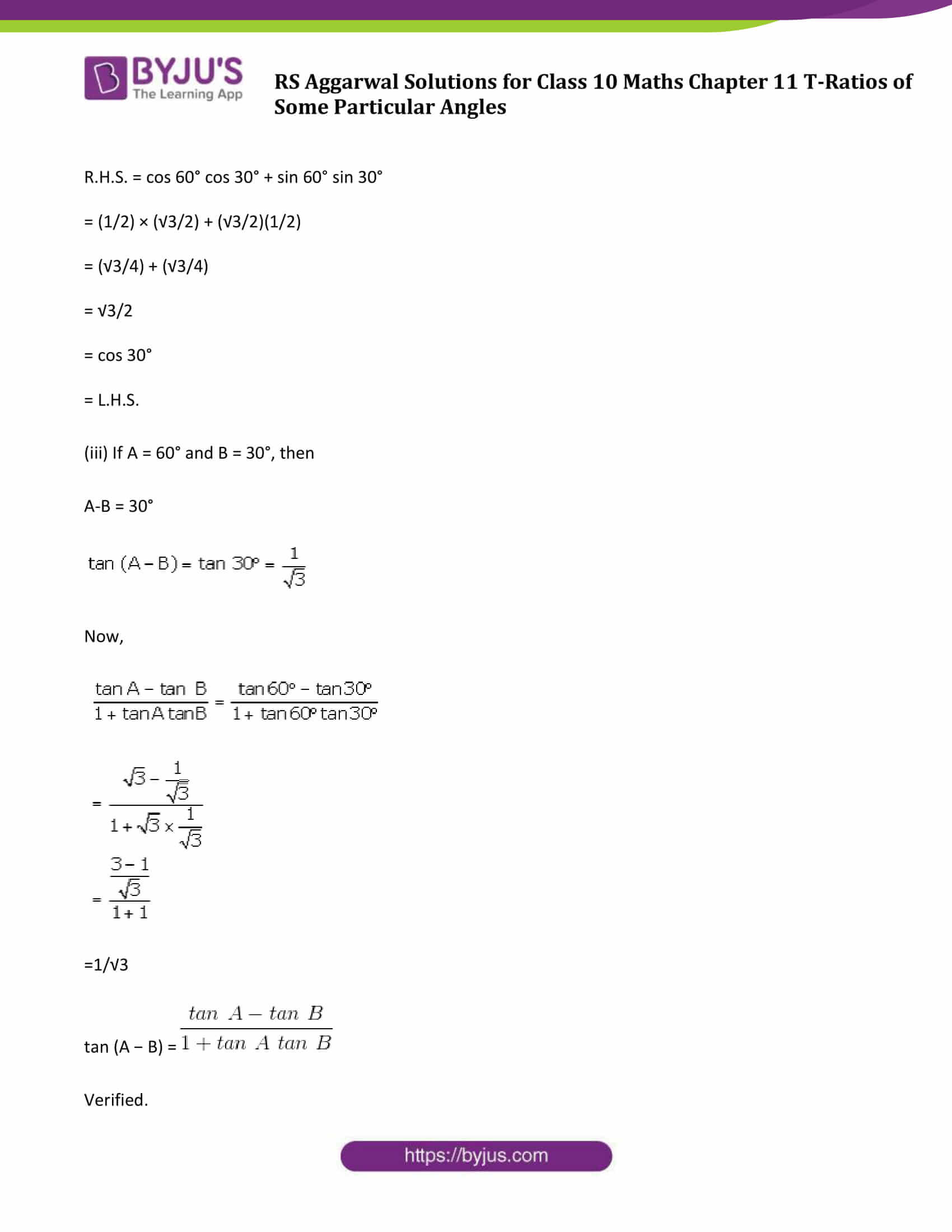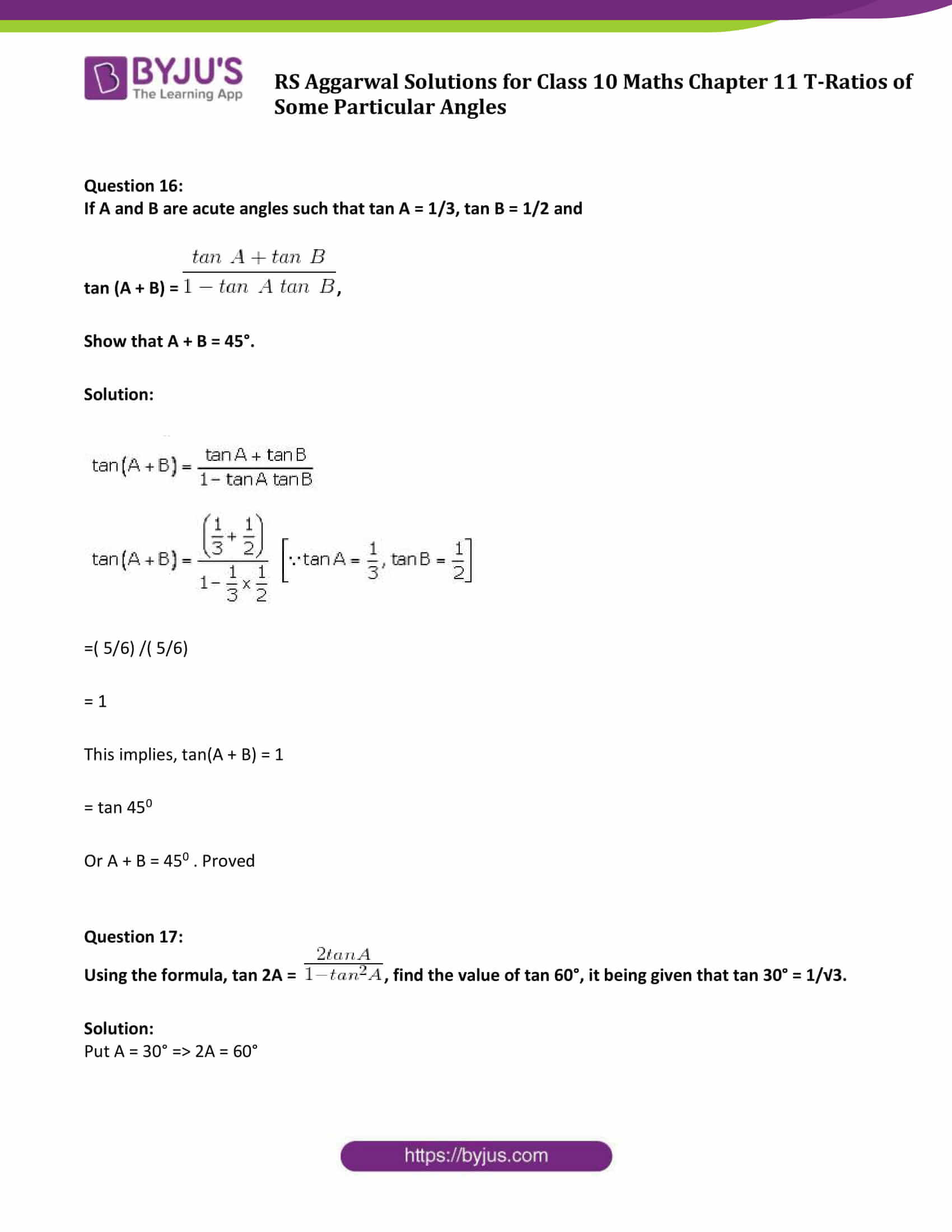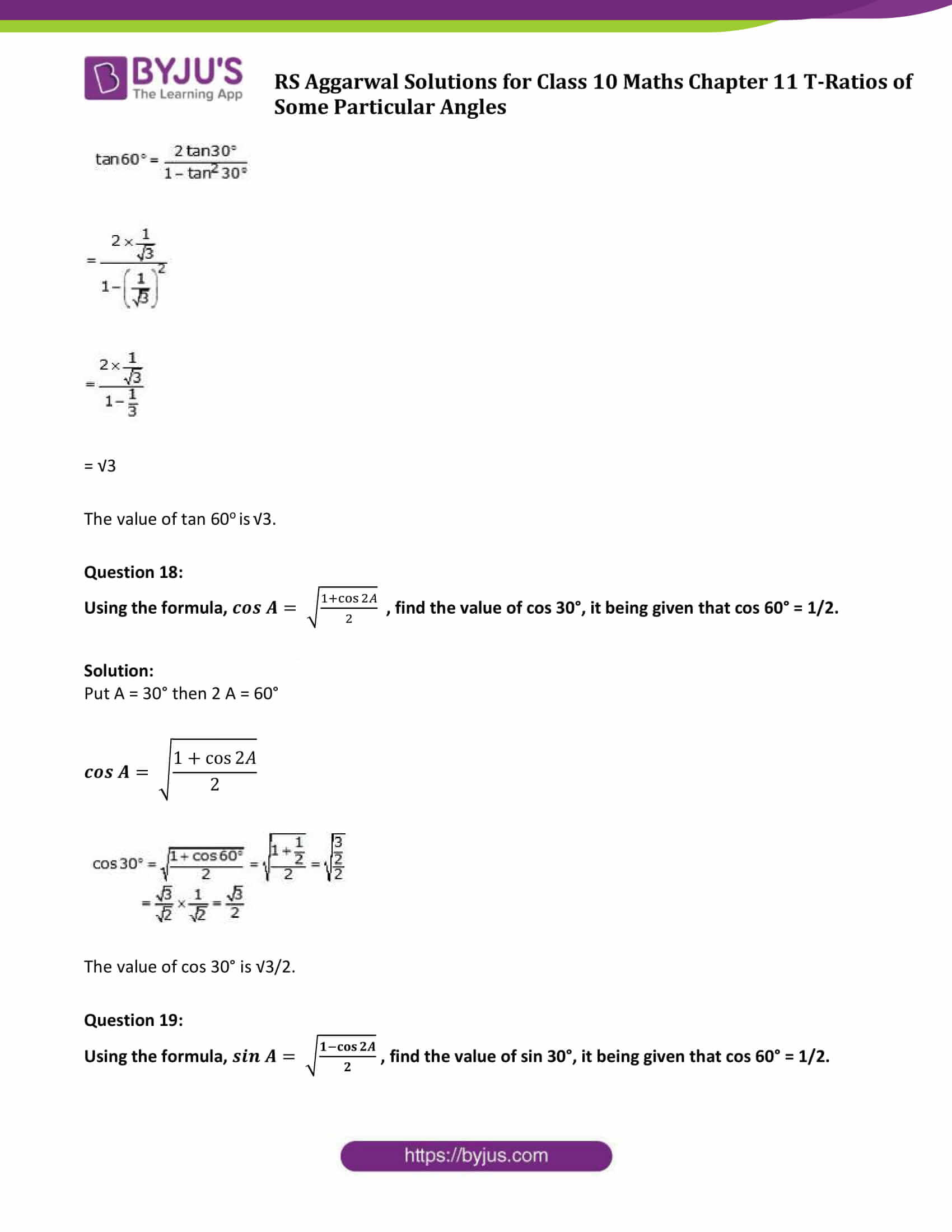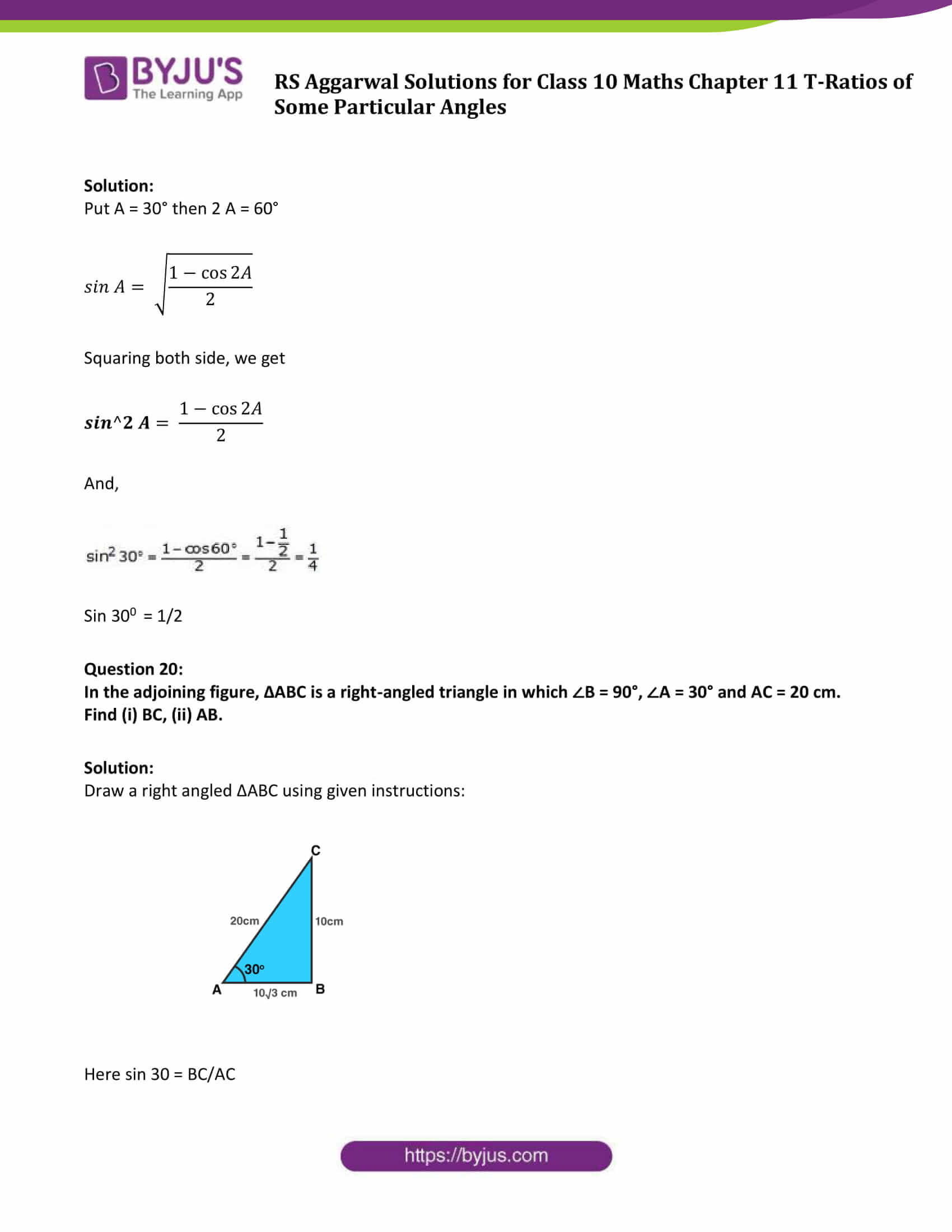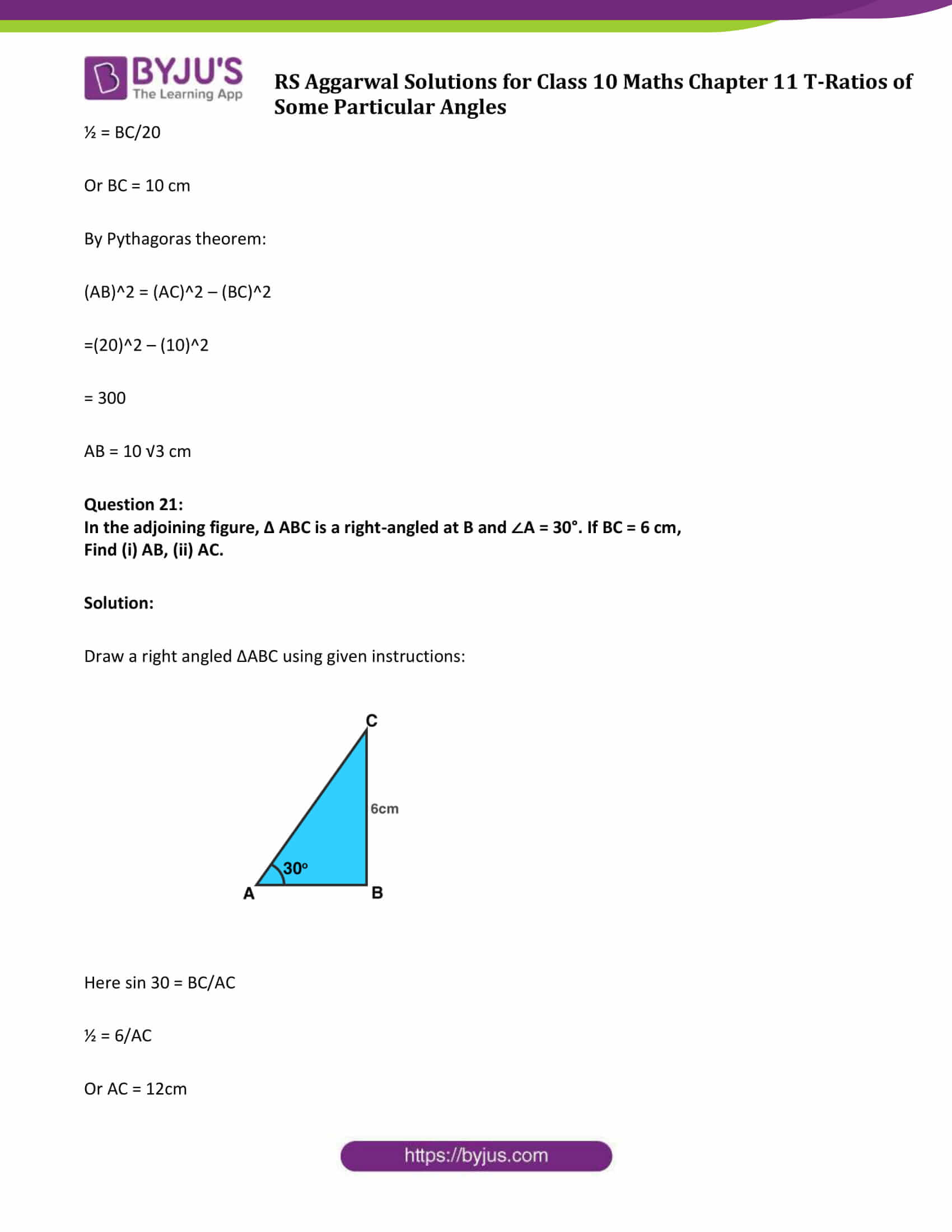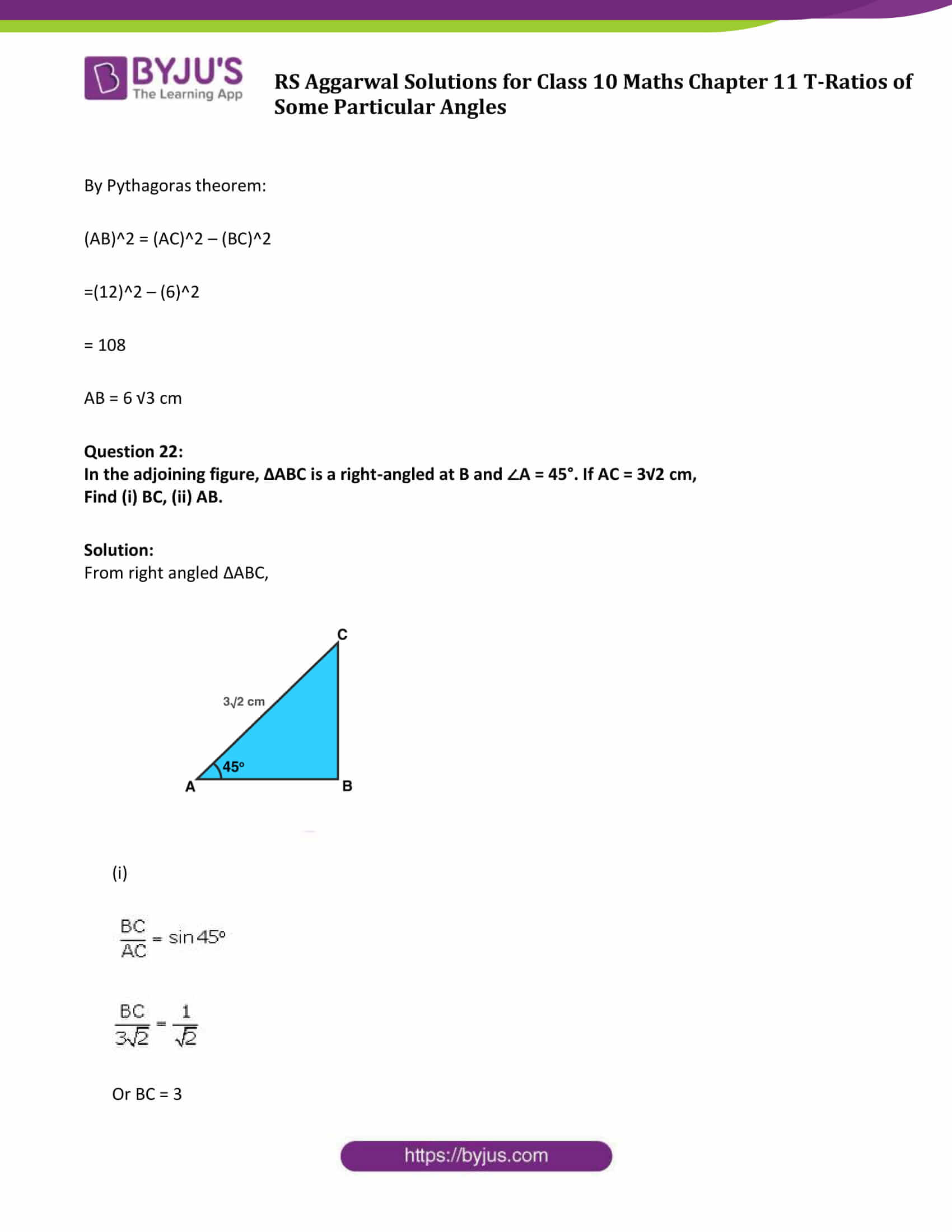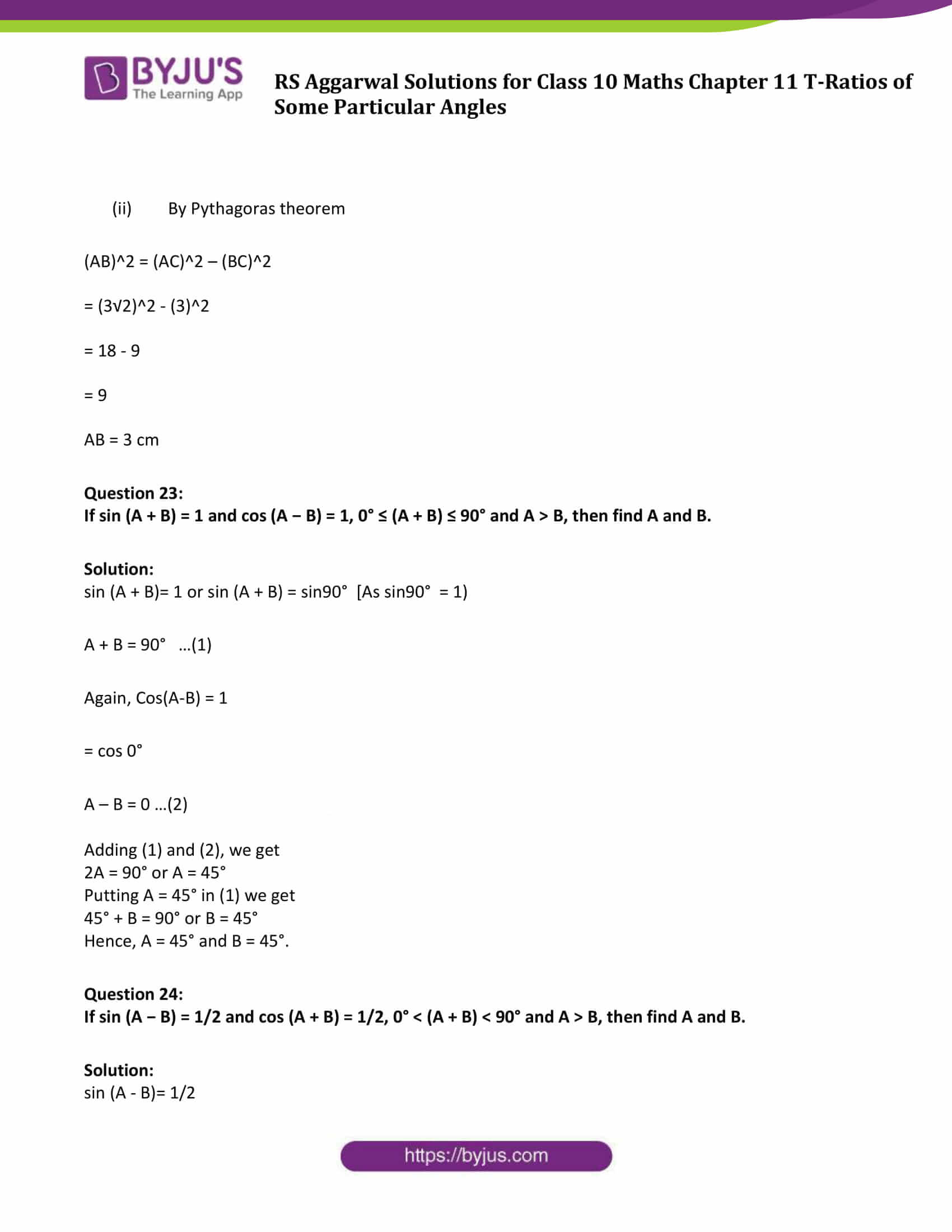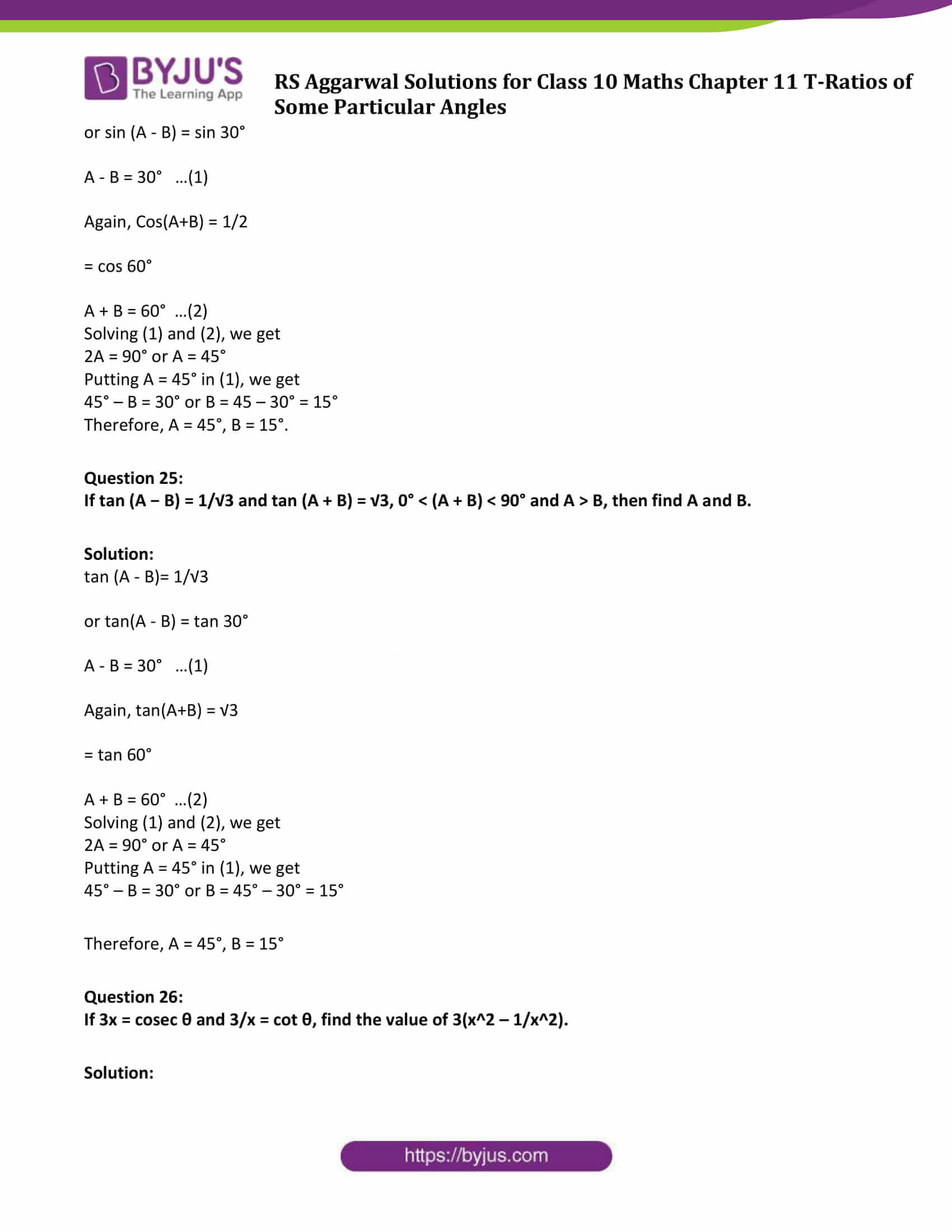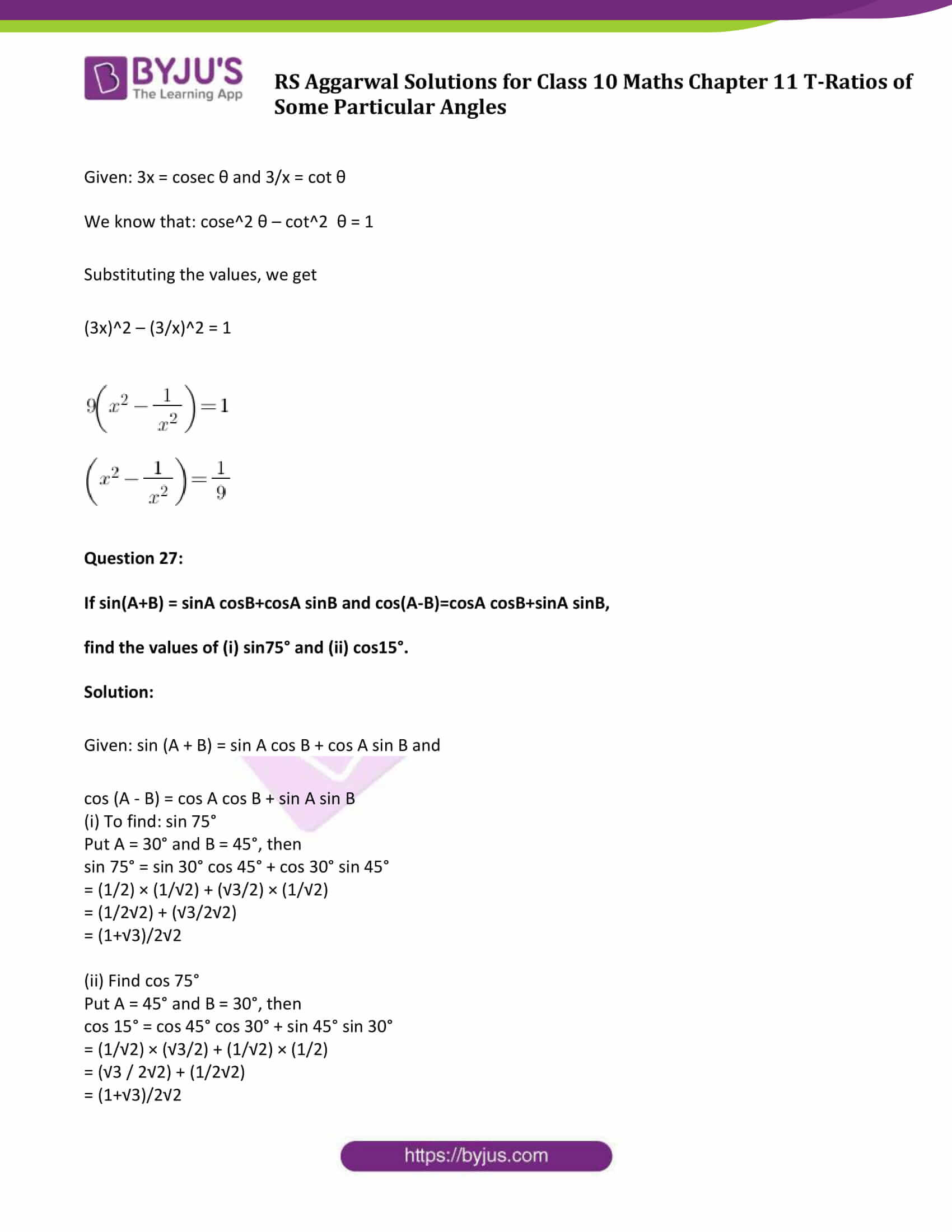### Access Answers to Maths R S Aggarwal Chapter 11 T-Ratios of Some Particular Angles Exercise 11

Evaluate each of the following:

(Question 1 to Question 9)

Question 1: sin 60° cos 30° + cos 60° sin 30°.

Solution:

We know that,

sin 60° = √3/2 = cos 30°

and sin 30° = 1/2 = cos 60°

Now,

sin 60° cos 30° + cos 60° sin 30° = (√3/2)( √3/2) + (1/2)(1/2)

= (3/4) + (1/4)

= 4/4

= 1

Question 2: cos 60° cos 30° − sin 60° sin 30°.

Solution:

We know that,

cos 60° = 1/2 = sin 30°

and cos 30° = √3/2 = sin 60°

Now,

cos 60° cos 30° — sin 60° sin 30° = (1/2) × (√3/2) – (√3/2) × (1/2)

= (√3/4) – (√3/4)

= 0

Question 3: cos 45° cos 30° + sin 45° sin 30°.

Solution:

We know that,

cos 45° = 1/√2 = sin 45°

cos 30° = √3/2 and

sin 30° = 1/2

Now,

cos 45° cos 30° + sin 45° sin 30° = (1/√2)×(√3/2) + (1/√2)(1/2)

= (√3 / 2√2) + (1 / 2√2)

= (√3 + 1) / (2√2)

Question 4: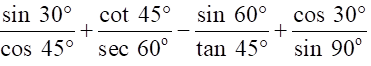Solution:

We know that,

sin 30° = 1/2 , sin 60° = √3/2 , sin 90° = 1

cos 30° = √3/2 , cos 45° = 1/√2 , cos 60° = 1/2

sec 60° = 2, tan 45° = 1 and cot 45° = 1

Now,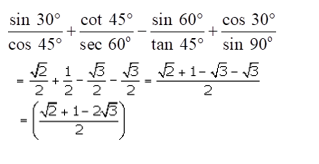Question 5: (5cos260°+4sec230°-tan245°)/(sin230°+cos230°)

Solution:

We know that,

cos 30° = √3/2 ⇨ cos2 30° = 3/4

cos 60° = 1/2 ⇨ cos2 60° = 1/4

sec 30° =(2/√3) ⇨ sec2 30° = 4/3

tan 45° = 1 ⇨ tan2 45° = 1

sin 30° = 1/2 ⇨ sin2 30° = 1/4

Now,

(5cos260°+4sec230°-tan245°)/(sin230°+cos230°)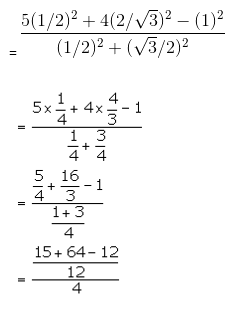= 67/12

Question 6:

2cos2 60° + 3sin2 45° − 3sin2 30° + 2cos2 90°.

Solution:

We know that,

sin 45° = 1/√2 , cos 60° = 1/2

sin 30° = 1/2 and cos 90° = 0

Now,

2cos2 60° + 3sin2 45° − 3sin2 30° + 2cos2 90°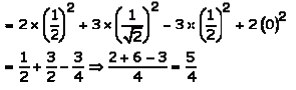Question 7:

cot230° − 2cos230° − ¾ sec245° + ¼ cosec230°.

Solution:

We know that,

Cot 30° = √3, cos 30° = √3/2

Sec 45° = √2 , cosec 30° = 2

Now,

cot230° − 2cos230° − ¾ sec245° + ¼ cosec230°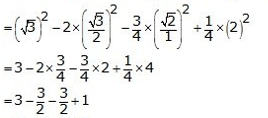= 1

Question 8:

(sin230° + 4cot245° − sec260°)(cosec245° sec230°).

Solution:

sin 30° = 1/2 ⇒ sin2 30° = 1/4

cos 45° = 1/√2 = sin 45°

cot 45° = 1 ⇒ cot2 45° = 1

cos 60° = 1/2 ⇒ sec 60° = 2 ⇒ sec2 60° = 4

cos 30° = √3/2 ⇒ sec 30° = 2/√3 ⇒ sec2 30° = 4/3

cosec 45° = 1/sin 45° = √2 ⇒ cosec2 45° = 2

(sin230° + 4cot245° − sec260°)(cosec245° sec230°)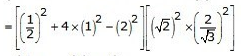=1/4 x 8/3

= 2/3

Question 9:

4/cot230°+1/sin230° – 2cos245°-sin20°.

Solution:

4/cot230°+1/sin230° – 2cos245°-sin2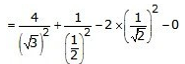= 4/3 + 4/1 – 1 – 0

= 26/6

= 13/3

Question 10:

Show that: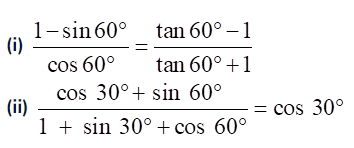Solution:

(i)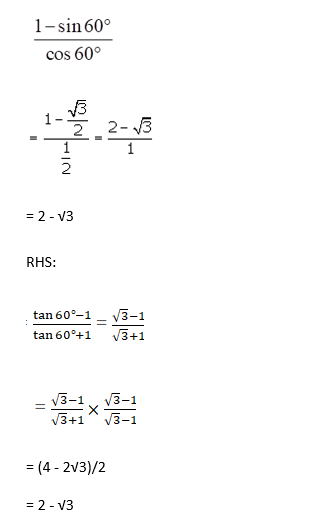(ii)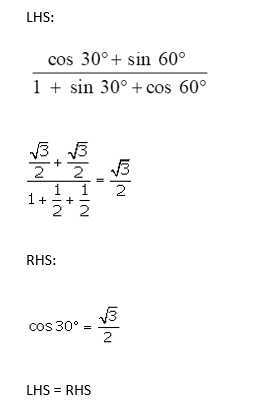Question 11:

Verify each of the following:

(i) sin 60° cos 30° − cos 60° sin 30° = sin 30°

(ii) cos 60° cos 30° + sin 60° sin 30° = cos 30°

(iii) 2 sin 30° cos 30° = sin 60°

(iv) 2 sin 45° cos 45° = sin 90°

Solution:

(i) L.H.S. = sin 60° cos 30° — cos 60° sin 30°

= (√3/2) × (√3/2) – (1/2)(1/2)

= (3/4) – (1/4)

= 2/4

= 1/2

R.H.S.:

sin 30° = 1/2

LHS = RHS

(ii)

L.H.S. = cos 60° cos 30° + sin 60° sin 30°

= (1/2) × (√3/2) + (√3/2)(1/2)

= (√3/4) + (√3/4)

= √3/2

R.H.S.

cos 30° = √3/2

L.H.S. = R.H.S.

(iii)

L.H.S. = 2 sin 30° cos 30°

= 2 × (1/2) × (√3/2)

= √3/2

R.H.S.

sin 60° = √3/2

L.H.S. = R.H.S.

(iv)L.H.S. = 2 sin 45° cos 45°

= 2 × (1/√2) × (1/√2)

= (2 × 1/2)

= 1

R.H.S. = sin 90° = 1

L.H.S. = R.H.S.

Question 12:

If A = 45°, verify that:

(i) sin 2A = 2 sin A cos A (ii) cos 2A = 2 cos2 A − 1 = 1 − 2 sin2 A

Solution:

A = 45° then 2 A = 90°

(i)Sin 2A = sin90°

RHS:

2 sin 45° cos 45° = 2 × (1/√2) × (1/√2)

= 1

LHS:

sin 90° = 1

L.H.S. = R.H.S.

(ii) cos 2A = cos90° = 0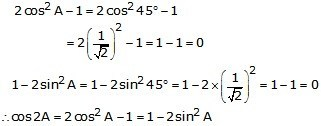Question 13.

If A = 30°, verify that: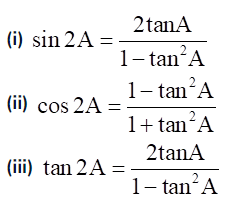Solution:

A = 30 ⇒ 2A = 60

(i)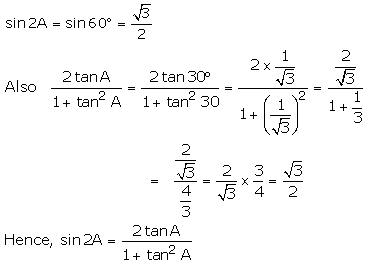(ii)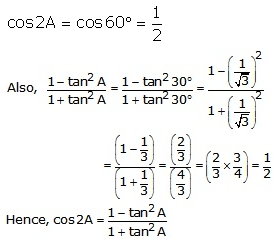(iii)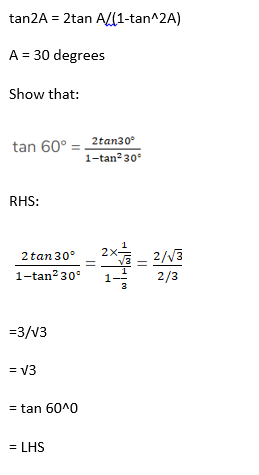Question 14:

If A = 60° and B = 30°, verify that:

(i) sin (A + B) = sin A cos B + cos A sin B

(ii) cos (A + B) = cos A cos B − sin A sin B

Solution:

(i) sin (A + B) = sin A cos B + cos A sin B

If A = 60° and B = 30°, then

To verify: sin 90° = sin 60° cos 30° + cos 60° sin 30°

RHS: sin 60° cos 30° + cos 60° sin 30°

= (√3/2) × (√3/2) + (1/2)(1/2)

= (3/4) + (1/4)

= 4/4

= 1

= sin 90°

= LHS

(ii) cos (A + B) = cos A cos B — sin A sin B

If A = 60° and B = 30°

Verify: cos (90°) = cos 60° cos 30° — sin60° sin 30°

R.H.S. = cos 60° cos 30° – sin 60° sin 30°

= (1/2) × (√3/2) – (√3/2)(1/2)

= (√3/4) – (√3/4)

= 0

= cos 90°

= L.H.S.

Question 15:

If A = 60° and B = 30°, verify that:

(i) sin (A − B) = sin A cos B − cos A sin

(ii) cos (A − B) = cos A cos B + sin A sin B

(iii) $tan(A-B) = \frac{tan A – tan B}{1 + tan A tan B}$

Solution:

(i) sin (A – B) = sin A cos B – cos A sin B

If A = 60° and B = 30°, then

LHS :

= sin(60°-30°)

= sin 30°

= 1/2

R.H.S. = sin 60° cos 30° – cos 60° sin 30°

= (√3/2) × (√3/2) – (1/2) (1/2)

= (3/4) – (1/4)

= 2/4

= 1/2

L.H.S. = R.H.S.

(ii) cos (A – B) = cos A cos B + sin A sin B

If A = 60° and B = 30°, then

Verify: cos (30°) = cos 60° cos 30° + sin 60° sin 30°

R.H.S. = cos 60° cos 30° + sin 60° sin 30°

= (1/2) × (√3/2) + (√3/2)(1/2)

= (√3/4) + (√3/4)

= √3/2

= cos 30°

= L.H.S.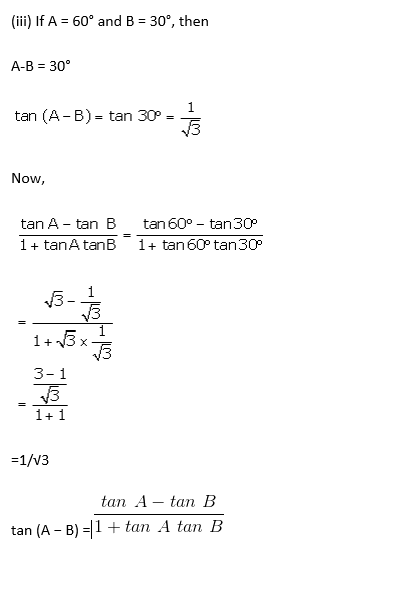Verified.

Question 16:

If A and B are acute angles such that tan A = 1/3, tan B = 1/2 and

$tan(A+B) = \frac{tan A + tan B}{1 – tan A tan B}$

Show that A + B = 45°.

Solution: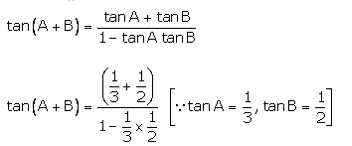=( 5/6) /( 5/6)

= 1

This implies, tan(A + B) = 1

= tan 450

Or A + B = 450 . Proved

Question 17:

Using the formula, $tan(2A) = \frac{2tan A}{1 – tan^{2} A }$ , find the value of tan 60°, it being given that tan 30° = 1/√3.

Solution:

Put A = 30° ⇨ 2A = 60°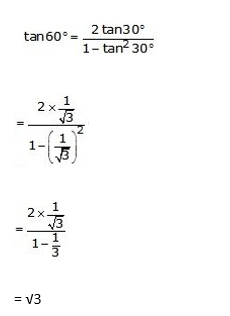The value of tan 60o is √3.

Question 18:

Using the formula, $cos A = \sqrt{\frac{1 + cos2A}{2}}$ , find the value of cos 30°, it being given that cos 60° = 1/2.

Solution:

Put A = 30° then 2 A = 60°

$cos A = \sqrt{\frac{1 + cos2A}{2}}$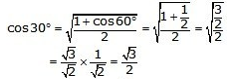The value of cos 30° is √3/2.

Question 19:

Using the formula, $sin A = \sqrt{\frac{1 – cos2A}{2}}$ , and the value of sin 30°, it being given that cos 60° = 1/2.

Solution:

Put A = 30° then 2 A = 60°

$sin A = \sqrt{\frac{1 – cos2A}{2}}$

Squaring both side, we get

$sin^{2} A = \frac{1 – cos2A}{2}$

And,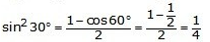Sin 300 = 1/2

Question 20:

In the adjoining figure, ∆ABC is a right-angled triangle in which ∠B = 90°, ∠A = 30° and AC = 20 cm.
Find (i) BC, (ii) AB.

Solution:

Draw a right angled ∆ABC using given instructions: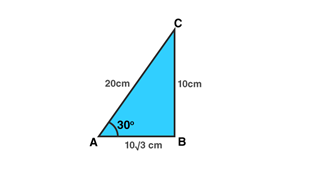Here sin 30 = BC/AC

½ = BC/20

Or BC = 10 cm

By Pythagoras theorem:

(AB)2 = (AC)2 – (BC)2

=(20)2 – (10)2

= 300

AB = 10 √3 cm

Question 21:

In the adjoining figure, ∆ ABC is a right-angled at B and ∠A = 30°. If BC = 6 cm,
Find (i) AB, (ii) AC.

Solution:

Draw a right angled ∆ABC using given instructions: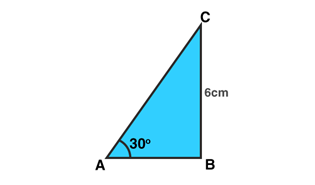Here sin 30 = BC/AC

½ = 6/AC

Or AC = 12cm

By Pythagoras theorem:

(AB)2 = (AC)2 – (BC)2

=(12)2 – (6)2

= 108

AB = 6 √3 cm

Question 22:

In the adjoining figure, ∆ABC is a right-angled at B and ∠A = 45°. If AC = 3√2 cm,
Find (i) BC, (ii) AB.

Solution:

From right angled ∆ABC,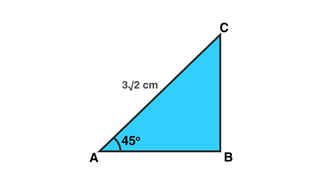1. BC/AC = sin45
$\frac{BC}{3\sqrt{2}} = \frac{1}{\sqrt{2}}$

Or BC = 3

1. By Pythagoras theorem

(AB)2 = (AC)2 – (BC)2

= (3√2)2 – (3)2

= 18 – 9

= 9

AB = 3 cm

Question 23:

If sin (A + B) = 1 and cos (A − B) = 1, 0° ≤ (A + B) ≤ 90° and A > B, then find A and B.

Solution:

sin (A + B)= 1 or sin (A + B) = sin90° [As sin90° = 1)

A + B = 90° …(1)

Again, Cos(A-B) = 1

= cos 0°

A – B = 0 …(2)

Adding (1) and (2), we get

2A = 90° or A = 45°

Putting A = 45° in (1) we get

45° + B = 90° or B = 45°

Hence, A = 45° and B = 45°.

Question 24:

If sin (A − B) = 1/2 and cos (A + B) = 1/2, 0° < (A + B) < 90° and A > B, then find A and B.

Solution:

sin (A – B)= 1/2

or sin (A – B) = sin 30°

A – B = 30° …(1)

Again, Cos(A+B) = 1/2

= cos 60°

A + B = 60° …(2)

Solving (1) and (2), we get

2A = 90° or A = 45°

Putting A = 45° in (1), we get

45° – B = 30° or B = 45 – 30° = 15°

Therefore, A = 45°, B = 15°.

Question 25:

If tan (A − B) = 1/√3 and tan (A + B) = √3, 0° < (A + B) < 90° and A > B, then find A and B.

Solution:

tan (A – B)= 1/√3

or tan(A – B) = tan 30°

A – B = 30° …(1)

Again, tan(A+B) = √3

= tan 60°

A + B = 60° …(2)

Solving (1) and (2), we get

2A = 90° or A = 45°

Putting A = 45° in (1), we get

45° – B = 30° or B = 45° – 30° = 15°

Therefore, A = 45°, B = 15°

Question 26:If 3x = cosec θ and 3/x = cot θ, find the value of 3(x2 – 1/x2).

Solution:

Given: 3x = cosec θ and 3/x = cot θ

We know that: cose2 θ – cot2 θ = 1

Substituting the values, we get

(3x)2 – (3/x)2 = 1

9( x2 – 1/ x2) = 1

( x2 – 1/ x2) = 1/9

Question 27:

If sin(A+B) = sinA cosB+cosA sinB and cos(A-B)=cosA cosB+sinA sinB,

find the values of (i) sin75° and (ii) cos15°.

Solution:

Given: sin (A + B) = sin A cos B + cos A sin B and

cos (A – B) = cos A cos B + sin A sin B

(i) To find: sin 75°

Put A = 30° and B = 45°, then

sin 75° = sin 30° cos 45° + cos 30° sin 45°

= (1/2) × (1/√2) + (√3/2) × (1/√2)

= (1/2√2) + (√3/2√2)

= (1+√3)/2√2

(ii) Find cos 75°

Put A = 45° and B = 30°, then

cos 15° = cos 45° cos 30° + sin 45° sin 30°

= (1/√2) × (√3/2) + (1/√2) × (1/2)

= (√3 / 2√2) + (1/2√2)

= (1+√3)/2√2

## R S Aggarwal Solutions for Chapter 11 T-Ratios of Some Particular Angles Topics

In this chapter students will study important concepts on T-Ratios of Some Particular Angles as listed below:

• T-Ratios of Some Particular Angles introduction
• Trigonometric ratios of 45 degrees
• Trigonometric ratios of 60 degrees and 30 degrees
• Axioms for T-ratios of 0 degrees
• Axioms for T-ratios of 90 degrees

### Key Features of R S Aggarwal Solutions for Class 10 Maths Chapter 11 T-Ratios of Some Particular Angles

1. The information provided in R S Aggarwal solutions for class 10 Maths Chapter 11 T-Ratios of Some Particular Angles is authentic and easy to understand.

2. Best study material for revision.

3. These solutions will be useful for various competitive exams.